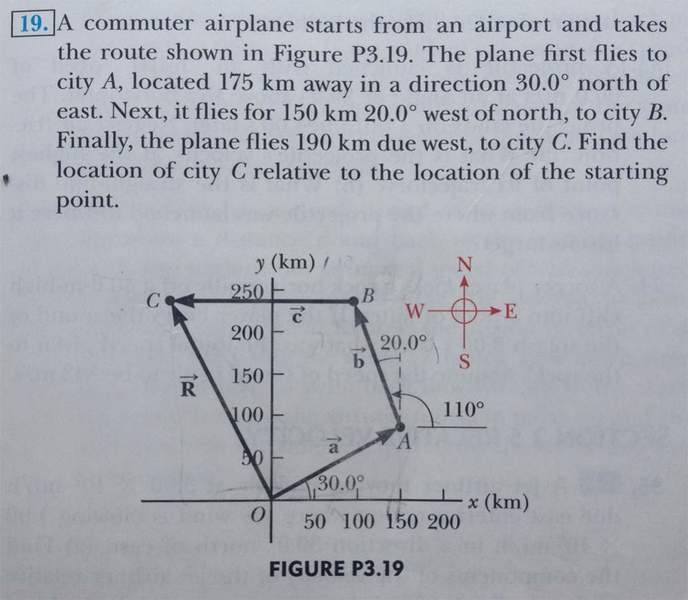# Adding vectors algebraically to find displacement

KevinFuerst
Hi everyone,
I am taking a physics course this year and I find myself already looking for help unfortunately. I think I understand the basics of adding vectors using Ax = A cosθ , Ay = A sinθ, etc. I am getting confused with the 3rd leg of the trip though. Since it is due west wouldn't that mean there is no vertical displacement? How can work that into my equation?

## Homework StatementAx = A cosθ
Ay = A sinθ
...
R = √Rx2 + Ry2

## The Attempt at a Solution

I have tried using the previous formulas to solve it but i get an answer of ≈320km. the back of the book says it should be 245km. could someone walk me though this? Thank you!

#### Attachments

Gold Member
What you want is $\vec{R} = \vec{a} + \vec{b} + \vec{c}.$
Write out the vector equations for each of $\vec{a}, \vec{b}, \vec{c}$ and then add them together. This will give an eqn for $\vec{R}$

Using $|\vec{R}| = \sqrt{R_x^2 + R_y^2}$ will give you it's length.
Can you find the angle from this?

KevinFuerst
thanks for the quick response but I am not quite following you guys. Still new to this.
Here is my work, maybe you can show me what i am doing wrong that way. Once i get the right distance i'll have no problem finding the angle. I under stand how to do that part.

Ax = A cos(30) = 175km (.866) = 151.55km
Ay = A sin(30) = 175 (.5) = 87.5km
Bx = B sin(20) =150km (.342) = -51.3km
By = B cos(20) = 150 (.939) = 140.96km
Cx = C cos(110) = -190km (-.342) = 64.98km
Cy = C sin(110) = 190 (.939) = 178.54km

√165.232 + 4072 = √192951.4 ≈ 439km

kushan
Hey kelvin ,
As the diagram and the question says , the vector C is parallel to X axis , which mean there is no change in the y component of total displacement .
On the side note , What does the figure mean by 110 degrees ? what is it .

kushan
Try solving
Cx as 190
and Cy as 0

Gold Member
Cx = C cos(110) = -190km (-.342) = 64.98km
Cy = C sin(110) = 190 (.939) = 178.54km

You have the components correct for vectors $\vec{a}$ and $\vec{b},$however the angles are wrong for the components of $\vec{c}$

What angle does $\vec{c}$ make with the positive $x$ direction?
There is no vertical component to $\vec{c}$

KevinFuerst
Try solving
Cx as 190
and Cy as 0
thank you so much. i understand that now. it will help me on a previos one i thought i had right too.

You have the components correct for vectors $\vec{a}$ and $\vec{b},$however the angles are wrong for the components of $\vec{c}$

What angle does $\vec{c}$ make with the positive $x$ direction?
There is no vertical component to $\vec{c}$

vector c is at 0 or 180 degrees isn't it?

kushan
180 you can call it .

Gold Member
Vector $\vec{c}$ is at angle of 180o with respect to the positive $x$ axis. Therefore the vector eqn for $\vec{c}$ is,
$\vec{c} = (190cos180)\hat x = -190\hat x$

KevinFuerst
oh okay! thats where that 110 came from. i was trying to figure out the angle so i decided to try the interior angle of ΔABC

i had a very similar problem there i found the interior angle like that and coincidently the resultant was extremely close either way. I have now corrected both and i cant thank you two enough.